# The GLIMMIX Procedure

#### The Odds Ratio Estimates Table

This table is produced by the ODDSRATIO option in the MODEL statement. It consists of estimates of odds ratios and their confidence limits. Odds ratios are produced for the following:

• classification main effects, if they appear in the MODEL statement

• continuous variables in the MODEL statement, unless they appear in an interaction with a classification effect

• continuous variables in the MODEL statement at fixed levels of a classification effect, if the MODEL statement contains an interaction of the two.

• continuous variables in the MODEL statements if they interact with other continuous variables

##### The Default Table

Consider the following PROC GLIMMIX statements that fit a logistic model with one classification effect, one continuous variable, and their interaction (the ODDSRATIO option in the MODEL statement requests the "Odds Ratio Estimates" table).

proc glimmix;
class A;
model y = A x A*x / dist=binary oddsratio;
run;


By default, odds ratios are computed as follows:

• The covariate is set to its average,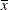, and the least squares means for the A effect are obtained. Suppose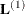denotes the matrix of coefficients defining the estimable functions that produce the a least squares means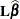, and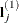denotes the jth row of. Differences of the least squares means against the last level of the A factor are computed and exponentiated: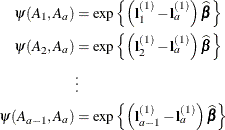The differences are checked for estimability. Notice that this set of odds ratios can also be obtained with the following LSMESTIMATE statement (assuming A has five levels):

lsmestimate A 1  0  0  0 -1,
0  1  0  0 -1,
0  0  1  0 -1,
0  0  0  1 -1 / exp cl;


You can also obtain the odds ratios with this LSMEANS statement (assuming the last level of A is coded as 5):

lsmeans A / diff=control('5') oddsratio cl;

• The odds ratios for the covariate must take into account that x occurs in an interaction with the A effect. A second set of least squares means are computed, where x is set to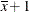. Denote the coefficients of the estimable functions for this set of least squares means as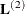. Differences of the least squares means at a given level of factor A are then computed and exponentiated: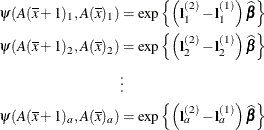The differences are checked for estimability. If the continuous covariate does not appear in an interaction with the A variable, only a single odds ratio estimate related to x would be produced, relating the odds of a one-unit shift in the regressor from.

Suppose you fit a model that contains interactions of continuous variables, as with the following statements:

proc glimmix;
class A;
model y = A x x*z / dist=binary oddsratio;
run;


In the computation of the A least squares means, the continuous effects are set to their means—that is,and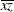. In the computation of odds ratios for x, linear predictors are computed at x =, x*z =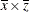and at x =, x*z =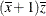.

##### Modifying the Default Table, Customized Odds Ratios

Several suboptions of the ODDSRATIO option in the MODEL statement are available to obtain customized odds ratio estimates. For customized odds ratios that cannot be obtained with these suboptions, use the EXP option in the ESTIMATE or LSMESTIMATE statement.

The type of differences constructed when the levels of a classification factor are varied is controlled by the DIFF= suboption. By default, differences against the last level are taken. DIFF=FIRST computes differences from the first level, and DIFF=ALL computes odds ratios based on all pairwise differences.

For continuous variables in the model, you can change both the reference value (with the AT suboption) and the units of change (with the UNIT suboption). By default, a one-unit change from the mean of the covariate is assessed. For example, the following statements produce all pairwise differences for the A factor:

proc glimmix;
class A;
model y = A x A*x / dist=binary
oddsratio(diff=all
at   x=4
unit x=3);
run;


The covariate x is set to the reference value x = 4 in the computation of the least squares means for the A odds ratio estimates. The odds ratios computed for the covariate are based on differencing this set of least squares means with a set of least squares means computed at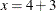.